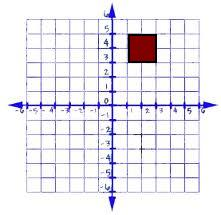# Identifying Translations Worksheets

How do you work with Geometric Translations? In geometric transformations, translation describes a function that moves or slides a subject to a certain distance. The shape, size, or measurements of the objects remains the same without being reflected, resized, or rotated in any way. When performing translation, we have to move every point of the object to the same distance. The initial object or the figure on which translation is being performed is known as the pre-image, and the figure obtained translation is known as the image. When we work with translation, the problem can be represented in different ways. You may get a figure presented on a graph; then, you will be given a direction and distance for translation.Let's work off of a cool hand drawing I just made on a napkin. High tech, I know... But the point is to show you how tangible these things are. For example, you are given a figure like the one drawn above. You are asked to perform translation down to 7. You will take each vertex and countdown to seven. So, for instance, the point present at (1,5) will shift to (1,-2). Note that you aren’t supposed to move the figure on the x-axis; you only have to move the figure on the y-axis (down to 7). Move the remaining vertices in the same way. You will get the figure at the following points: - (1,3) will shift to (1,-4), (3,5) will shift to (3,-2), (3,3) will shift to (3,-4).

• ### Basic Lesson

Guides students through the determination of a translation. The original object and its translation have the same shape and size. The original object and its translation face in the same direction.

• ### Intermediate Lesson

Demonstrates how to find the presence of a translation. Yes, this is translation because the object has same shape, size, and faces the same direction.

• ### Independent Practice 1

A really great activity for allowing students to reinforce the concept of Identifying Translations. For each problem, determine if it is an example of translation.

• ### Independent Practice 2

Students draw and Identifying Translations in assorted problems. The answers can be found below.

• ### Homework Worksheet

Students are provided with problems to achieve the concepts of Identifying Translations.

• ### Skill Quiz

This tests the students ability to master Identifying Translations.

Answers for the homework and quiz.

• ### Answer Key Part 2

Answers for lessons and both practice sheets.

• ### Basic Lesson

Guides students through the uses of translations. Under the translation (x + 2, y - 2), the point (3, 4) will become (5, 2).

• ### Intermediate Lesson

Demonstrates the how to determine is a translation occurred. Each point has same distance. A translation "slides" an object a fixed distance in a given direction. The original object and its translation have the same shape and size, and they face in the same direction.

• ### Independent Practice 1

A really great activity for allowing students to understand the concepts of the Translations.

• ### Independent Practice 2

Students use Translations in 20 assorted problems. The answers can be found below.

• ### Homework Worksheet

Students are provided with 12 problems to achieve the concepts of Translations.

• ### Skill Quiz

This tests the students ability to understand translations.